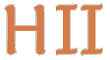# Hilbert II

 English Deutsch
QEDEQ Module

The mathematical knowledge of this project is organized in so called QEDEQ modules. Such a module can be read and edited with a simple text editor. It could contain references to other QEDEQ modules which lay anywhere in the world wide web.

A QEDEQ module is build like a mathematical text book. It contains chapters which are composed of paragraphs each with an axiom, abbreviation, definition or proposition. Every paragraph has a label and could be referenced by that label. Essential formal element of a paragraph are formulas. The formulas are written in a first order predicate calculus, also the proofs are in this language. Therefore a proof verifier can check the formulas and their proofs for formal correctness. We can create linked mathematical text books. And with the extended analytic possibilities of the formal language we can easily do for example a dependency analysis.

In addition to the basic rules also other derived rules, so called meta rules, could be used. A proof that uses meta rules could be automatically transformed into a proof which only uses the basis rules. Some other language extensions, for example abbreviations, are established for shorter writing and convenient argumentation. These extensions can also be automatically removed and transformed into the original system.
We are aware of the fact that this transformation is not in each case practically realisable. For example it is not possible to write down the natural number 1000000000000000 completely in set notation: {{}, {{}}, {{}, {{}}}, {{}, {{}}, {{}, {{}}}}, ...}.

The comprehension of mathematics is not promoted by formal languages. Hence descriptive texts written in the "colloquial language LaTeX" are of great importance. Lastly those texts carry the mathematical contents for humans. In the QEDEQ moduls of Hilbert II those texts are regular parts. There can also be different detail levels of texts and proofs. The first levels should be non formal proofs but common mathematical texts like "trivial", "follows directly from definition" or something more elaborated. Then the highest levels are formal correct proofs. It is also possible to give different proofs, for instance an elegant short one using the foundation axiom and a long and laborious one without the foundation axiom.

Out of the QEDEQ module hyperlinked LaTeX, HTML or PDF documents can be generated. These documents look basically like a common mathematical document. Before the generation the wanted detail level must be given.

The current QEDEQ format is an XML schema specification and is documented here.

In Logical Language more details about the formal formula and term syntax are explained. Here are also the basic formal proof rules listed.

See Elements of Mathematical Logic for the logical background of this project.

For the prototype another proprietary format is used.

update 2014-01-20 09:20:48+0100